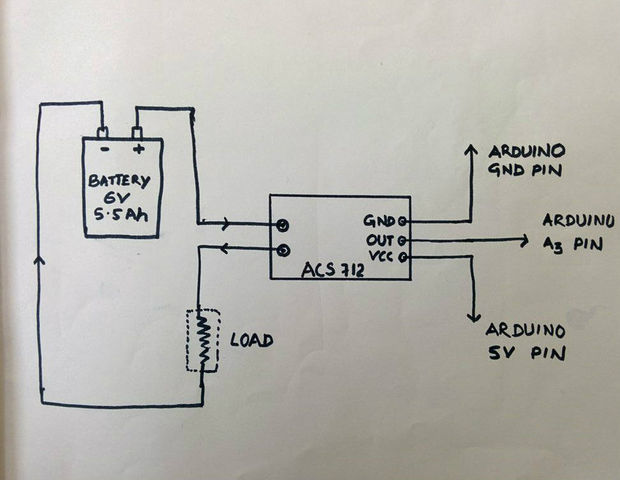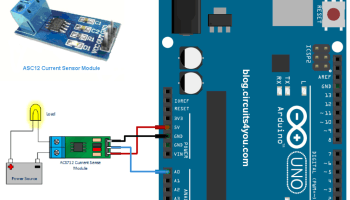# Current measurement problem with ACS712 Current Sensor (30A)

I am try to measure DC current with ACS712 current sensor but it was not showing current.

i am using Arduino nano.

``````/*
Measuring Current Using ACS712
www.circuits4you.com
*/
double mVperAmp = 66; // use 100 for 20A Module and 66 for 30A Module
double RawValue= 0;
double ACSoffset = 2500;
double Voltage = 0;
double Amps = 0;

void setup(){
Serial.begin(9600);
}

void loop(){
Voltage = (RawValue / 1024.0) * 5000; // Gets you mV
Amps = ((Voltage - ACSoffset) / mVperAmp);

Serial.print("Amps = "); // shows the voltage measured
Serial.println(Amps,2); // the '2' after voltage allows you to display 2 digits after decimal point
delay(1000);
}
``````

Does this display anything?
(uncompiled, untested)

``````const byte analogIn = A0;
const float mVperAmp = 66; // use 100 for 20A Module and 66 for 30A Module
const float ACSoffset = 2500;

void setup()
{
Serial.begin(9600);
}

void loop(){
float Voltage = (RawValue / 1024.0) * 5000.0; // Gets you mV
float Amps    = ((Voltage - ACSoffset) / mVperAmp);

Serial.print(F("Amps = "));
Serial.println(Amps);
delay(1000);
}
``````
2. change declaration of mVperAmp to 66.0 (I'm guessing your amps is getting converted to an integer
3. write the RawValue right after you read it--i'd be curious to see if you are reading anything

Hope this helps

it was showing same.KrisKasprzak:

2. change declaration of mVperAmp to 66.0 (I'm guessing your amps is getting converted to an integer
3. write the RawValue right after you read it--i'd be curious to see if you are reading anything
1. Nope. It's an analogue input pin, read with an analogRead - why would you also want to make it a digital input? (which'll be overridden in the analogRead)
2. Nope. You're assigning it to a "double" (aka float) - the compiler is smart enough to do the conversion.
3. Reasonable.

One thing I like to do with any code like this is first print a "version message" or something similar to the display to verify the display is working as expected. Then, during the debug stage, always print the raw data first then your calculated data - that gives you a quick way to determine if you are reading garbage or creating it in your calculations. Once it all works as expected, comment out the raw data print statement. The trick when debugging is to break it down into chunks and figure which "chunk" is where the problem is (goes with good coding too).

``````/*
Measuring Current Using ACS712
www.circuits4you.com
*/
double mVperAmp = 66; // use 100 for 20A Module and 66 for 30A Module
double RawValue= 0;
double ACSoffset = 2500;
double Voltage = 0;
double Amps = 0;

void setup(){
Serial.begin(9600);
}

void loop(){
Voltage = (RawValue / 1024.0) * 5000; // Gets you mV
Amps = ((Voltage - ACSoffset) / mVperAmp);

Serial.print("Amps = "); // shows the voltage measured
Serial.println(Amps,2); // the '2' after voltage allows you to display 2 digits after decimal point
delay(1000);
}
``````

it was showing -0.15 and -0.22

One question?
ACS712 measure current from positive side or negative side.it was working with AC supply.

``````/*
Measuring AC Current Using ACS712
www.circuits4you.com
*/
const int sensorIn = A0;
int mVperAmp = 66; // use 100 for 20A Module and 66 for 30A Module

double Voltage = 0;
double VRMS = 0;
double AmpsRMS = 0;

void setup(){
Serial.begin(9600);
}

void loop(){

Voltage = getVPP();
VRMS = (Voltage/2.0) *0.707;  //root 2 is 0.707
AmpsRMS = (VRMS * 1000)/mVperAmp;
Serial.print(AmpsRMS);
Serial.println(" Amps RMS");
}

float getVPP()
{
float result;
int maxValue = 0;          // store max value here
int minValue = 1024;          // store min value here

uint32_t start_time = millis();
while((millis()-start_time) < 1000) //sample for 1 Sec
{
// see if you have a new maxValue
{
/*record the maximum sensor value*/
}
{
/*record the minimum sensor value*/
}
}

// Subtract min from max
result = ((maxValue - minValue) * 5.0)/1024.0;

return result;
}
``````

it was showing -0.15 and -0.22

With a multimeter:
What is the load resistance (ohms)? (R)
What is the DC votage drop measured across the load? (V)

Amps = V/R

Compare this to what you're measuring with the Arduino.

it was working with AC supply.

Are you sure your "read for 1 second" approach is valid? minValue will almost certainly be 0 every time I would think as you are bound to read at the zero crossing (or near it) at least once, unless...

...your read frequency inside getVPP() could "beat" with the mains frequency which would give you skewed results that would be different every time you called getVPP(). Unlikely with a fast loop I suppose, but how long does analogRead() take? What if it takes 20mS and that's the period of the AC wave form.

The code in post#0 seems fine for testing.
OP did not mention the test current through the sensor.
-0.22 or -0.15 without, or with a small current seems normal.

Sensors like this, measured with an Arduino, have the 30Amp span spread out over ~450 A/D values.
The setup should work, but zeroing without current, and measuring to 0.1Amp requires better code.

Try sending a few Amps DC through the sensor.
Leo..

Yes, right

I was giving more then 1A then it was measuring.

Now, I want to measure voltage also and display on 16*2 LCD.

Voltage 0-50V

anybody help me...